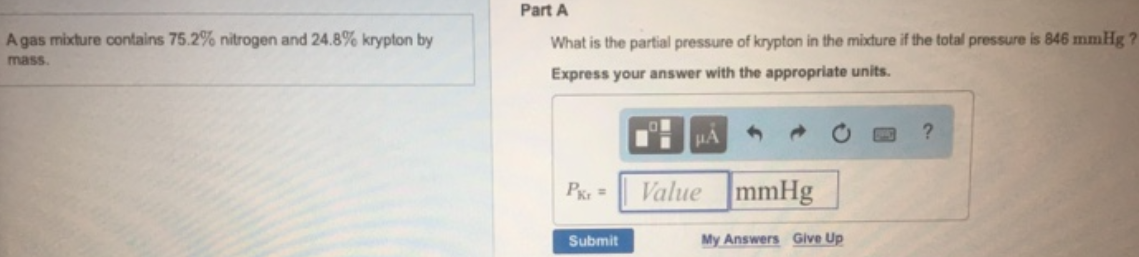# Problem: A gas mixture contains 75 2% nitrogen and 24 krypton by mass.What is the partial pressure of krypton in the mixture if the total pressure is 846 mmHg ? Express your answer with the appropriate units.

###### FREE Expert Solution###### Problem Details

A gas mixture contains 75 2% nitrogen and 24 krypton by mass.

What is the partial pressure of krypton in the mixture if the total pressure is 846 mmHg ?